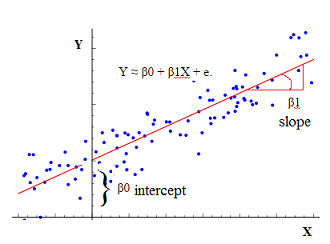# Statistics - Little r - (Pearson product-moment Correlation coefficient)

The Pearson product-moment correlation coefficient is a correlation coefficient formulas that can be applied when both variables are continuous.

## Syntax

$$\begin{array}{rrc} \text{little r} & = & \frac{\text{degree to which X and Y vary together}}{\text{degree to which X and Y vary independently}} \\ & = & \frac{\href{Covariance}{Covariance} \text{ of X and Y}}{\href{Variance}{Variance}\text{ of X and Y}} \end{array}$$

The correlation is the standardized Covariance as standard deviation is the standardized variance. (Standardized to get the value in the range).

## Formula

The raw score formula and the Z score formula gives the same result.

### Raw score

$$r = \frac\sum_{i=1}^{N}{\href{cross_product}{\text{Cross Product of X & Y}}}}\sqrt{ \sum_{i=1}^{N}{(\href{deviation_score}{\text{Deviation score of X}})^2} . \sum_{i=1}^{N}{(\href{deviation_score}{\text{Deviation score of Y}})^2} } }$$

where:

### Z-score

$$r = \frac\sum_{i=0}^{N}{ (\href{z_score}{\text{Z Score of X }})( \href{z_score}{\text{Z Score of Y}}) } } {N}$$

where:

Recommended PagesR - Correlation

with: confidence interval df (degree of freedom) t-value p-value cor (Pearson's product-moment correlation)R - Scatterplot

Useful way to see all plots for a two-class variable Standard scatterplot Standard scatterplot with regression line Scatterplot matrix Color scatterplot matrix, colored and ordered by...Statistics - (Univariate|Simple|Basic) Linear Regression

A Simple Linear regression is a linear regression with only one predictor variable (X). Correlation demonstrates the relationship between two variables whereas a simple regression provides an equation...Statistics - Correlation (Coefficient analysis)

Correlation is a statistical analysis used to measure and describe the relationship betweentwo variables. The Correlations coefficient is a statistic and it can range between +1 and -1 +1 is a perfect...Statistics - Correlation Matrix

From a raw matrix to a correlation matrix. A correlation matrix is a special matrix used in statistics. It is a square symmetric matrix. 3 columns (3 variables), 8 rows (8 individuals) ...Statistics - Cross Product (of X and Y) (CP|SP)

The cross product is a calculation used in order to define the correlation coefficient between two variables. SP is the sum of all cross products between two variables. For one observation (one...Statistics - Null Hypothesis Significance Testing (NHST)

NHST is a procedure for testing the Null Hypothesis. It's a binary decision: Reject or Retain the Null Hypothesis Ie Retain the Null Hypothesis or the alternative hypothesis. Before starting any...Statistics - R (Big R)

R (Big R) is a complex model with multiple correlation coefficient. R is an accuracy statistic for the overall model. R is: the correlation between the predicted scores and the observed scores R...